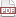### Transforming models

02 July 13. [link]PDF version

The story so far is that we've developed what mathematicians would call a category and what CompSci majors would call an object to represent a statistical model.

With this definition, we can sensibly write down transformations, in which we take every element of the model, and transform it to represent a new model. My go-to example is truncation. You start with a Normal distribution, and want a Normal distribution truncated so that all data values are greater than zero.

$\def\Re{{\mathbb R}} \def\datas{{\mathbb D}} \def\params{{\mathbb P}} \def\models{{\mathbb M}} \def\mod#1{M_{#1}}$

A model as defined last time consists of a data space, a parameter space, and four functions. The data space is cut to be greater than zero, the parameter space is unchanged (although $\mu < 0$ can be proven to be impossible), and we can tick down the list of functions and how we would need to wrap them. Given a Normal distribution object with $L_{\cal N}$, $RNG_{\cal N}$, $CDF_{\cal N}$, and $Est_{\cal N}$ methods, it would not be challenging to write a function that transforms that into a truncated Normal object. And I'm just using the Normal as an example: once we've written the truncation operator, it should be applicable to any properly black-boxed model.

For contrast, consider a system where a model is defined as a free list of functions, which can be arbitrarily extended by the model author. Then we wouldn't be able to write this transformation function, because we wouldn't know what is on the list of methods that have to be transformed.

In the paper this is based on, I brainstorm several additional transformations, many largely mechanical:

• data constraints, as above
• parameter constraints
• fixed parameters,wherein we turn a ${\cal N}(\mu, \sigma)$ into a ${\cal N}(\mu, 1)$
• stacking independent models
• mixtures of models
• jacobian transformations
• swapping parameters and data
• joining outputs from one model to inputs to the next (to be discussed in a few episodes)

Letting the set of models be $\models$, all of these map from $\models \to\models$ or $\models\times \models \to\models$, meaning that after the tranformation you have a model like any other, with some set of parameters, data, and the same functions ($L$, $Est$, $RNG$, $CDF$) that map between them. If you have a function that takes in a model (maybe a bootstrap or K-L divergence to other models), it can take in the output from one of these transformations.

Further, the mappings chain together. Given a data-constraint function $Constr:\models \to \models$, we could constrain a Normal distribution, producing the model $\mod{constr} = Constr(\mod{\cal N})$, then stack it on top of an indepented Poisson model to produce the model $\mod{pair} = Stack(\mod{constr}, \mod{poisson})$. Given an appropriate data set $D$, we are guaranteed that all of the methods of the composite model work: let $P = Est_{pair}(D)$, then we can make random draws $RNG_{pair}(P)$, or test hypotheses using $CDF_{pair}(\cdot, P)$.

At this point, we've already built a formidable language for modeling. Real-world processes tend to have some sort of narrative around them: maybe there's an unknown initial distribution of agents, and then some of them go left, and some go right, and those that went left get filtered in another manner, different from how the ones that went right got filtered. In the paper, I give the example of a dinner party, where some people try to show up fashionably late and some people try to be on time but hit an unknown number of delays. Nobody is early. I propose modeling this arrival time narrative using a mixture of a truncated Normal and a Jacobian-transformed Exponential distribution [$\mod{Arrival} = Mix(Trunc_{x>0}(\mod{\cal N}), Jacobian_{1/\lambda}(\mod{Exp}))$]. We've reduced the problem of developing a narrative model for a relatively complex and nuanced situation into a question of clicking together Legos.

It saddens me when I read papers where a theorist develops a detailed narrative model, and then tests it using a linear regression that is intended to approximate the narrative. Because the language of taking basic models and transforming them or joining them to produce complex models mirrors real-world narratives directly, it raises the odds that we can test claims directly against the narrative.

Next on the agenda: an example, Bayesian updating and hierarchical models, and some computational epistemology questions.